library(swimplot)
library(ggplot2)
#> Warning: package 'ggplot2' was built under R version 4.0.3

Introduction to swimmers plots

A swimmer plot is a graphical tool used to display individual trajectories over time.

A swimmer plot is able to tell a full story using horizontal bars to represent each subject (or study unit), while lines, points, and arrows are utilized to display additional information.

The “swimmer” package has a variety of functions which add layers to a swimmer plot by implementing ggplot functions.

This vignette goes through examples to create swimmers plots, and demonstrates converting a dataframe to the required format.

A working example

The Data and research question

• This is a clinical trial of 36 patients in which patients are randomized to one of two treatment arms, at 5 months patients are intended to switch arms, for each patient the adverse events and response information is recorded. The data is stored in three dataframes, ClinicalTrial.Arm, ClinicalTrial.AE, and ClinicalTrial.Response
id Arm End_trt Continued_treatment Sex Age
1 Arm A 3.26 NA F >=65
2 Arm A 2.00 NA F <65
2 Off Treatment 10.00 NA F <65
2 Arm A 15.45 NA F <65
3 Arm B 5.00 NA F >=65
3 Arm A 14.84 NA F >=65
4 Arm B 3.51 NA F <65
5 Arm B 6.00 NA F >=65
5 Arm A 7.44 NA F >=65
6 Arm B 3.70 NA F <65
id time event Related Sex Age
33 2.20 AE Likely M >=65
14 1.00 SAE Possibly F <65
14 3.67 Death Not Likely F <65
3 14.58 AE Likely F >=65
29 5.44 SAE Possibly M >=65
21 0.50 AE Possibly M >=65
10 5.00 SAE Not Likely F <65
10 6.06 SAE Possibly F <65
2 2.00 SAE Not Likely F <65
3 14.84 Death Likely F >=65
id Response_start Response_end Response Continued_response Sex Age
10 3.74 6.02 CR NA F <65
11 1.48 2.21 PR NA F >=65
12 0.20 0.67 CR NA F <65
13 2.07 2.59 CR NA F >=65
15 0.35 4.05 PR NA M >=65
16 4.49 7.30 CR NA M <65
17 1.09 1.44 PR NA M >=65
18 0.55 1.32 CR NA M <65
19 0.00 0.96 PR 1 M >=65
2 3.36 8.77 PR NA F <65

Basic plot

• The swimmer_plot() function creates the base of the swimmer plot
• The required arguments are a dataframe, an id column name, and the column name of where the bars end
• By default the bars are in increasing order, but any order can be specified
• A column name for the fill, transparency and colour (outline of the bars) can also be included
• Individual bars can change colour/transparency over time
• Other aesthetics can be manipulated using geom_bar() arguments (eg. fill,width, alpha)
swimmer_plot(df=ClinicalTrial.Arm,id='id',end='End_trt',fill='lightblue',width=.85)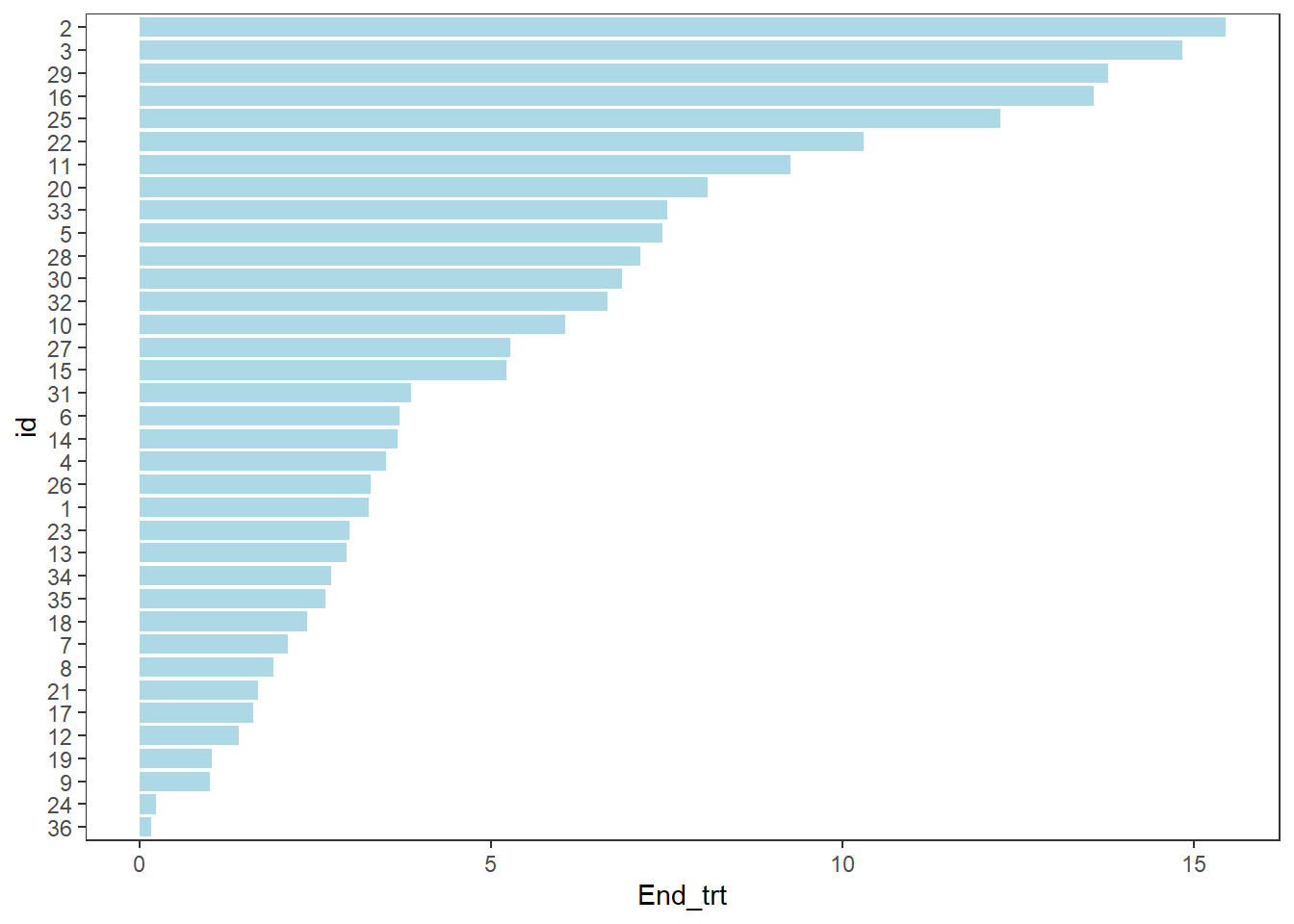Modifying the order and colours of the bars

The swimmer_plot() function includes the option to have the bars change colours. Each section of the bars should be in a different row, where each row includes the time that section ends. By default the bars are plotted in increasing order, a column name can be used in the argument id_order to have the bars sorted first by a column, or string of IDs can be specified to have the bars in a specific order. Here the bars are ordered by the starting treatment, and follow up time.

arm_plot <- swimmer_plot(df=ClinicalTrial.Arm,id='id',end='End_trt',name_fill='Arm',
id_order='Arm',col="black",alpha=0.75,width=.8)

arm_plot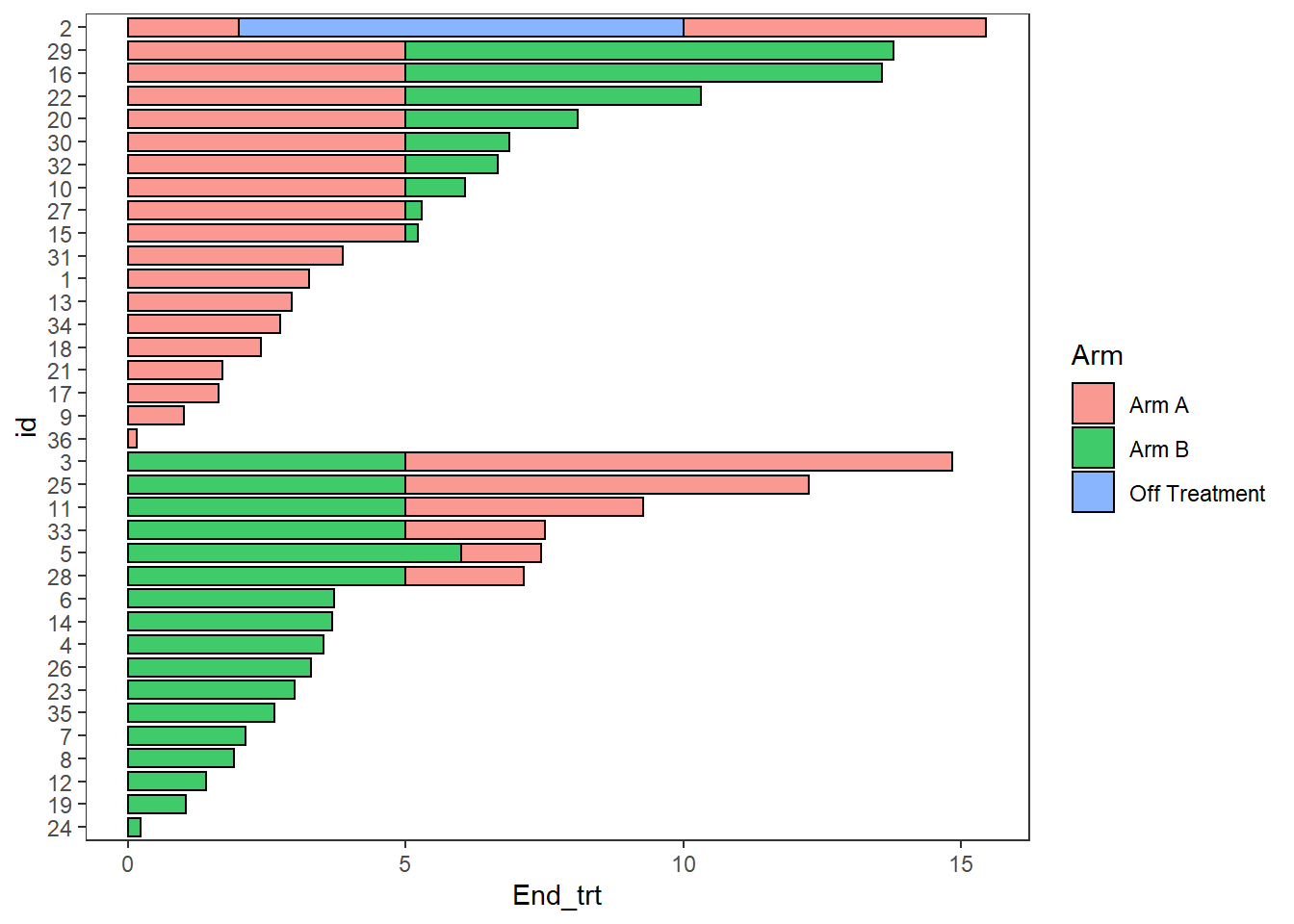Stratification

Plots can be stratified by any variables in the dataframe

swim_plot_stratify <-swimmer_plot(df=ClinicalTrial.Arm,id='id',end='End_trt',name_fill='Arm',
col="black",alpha=0.75,width=.8,base_size = 14,stratify= c('Age','Sex'))

swim_plot_stratify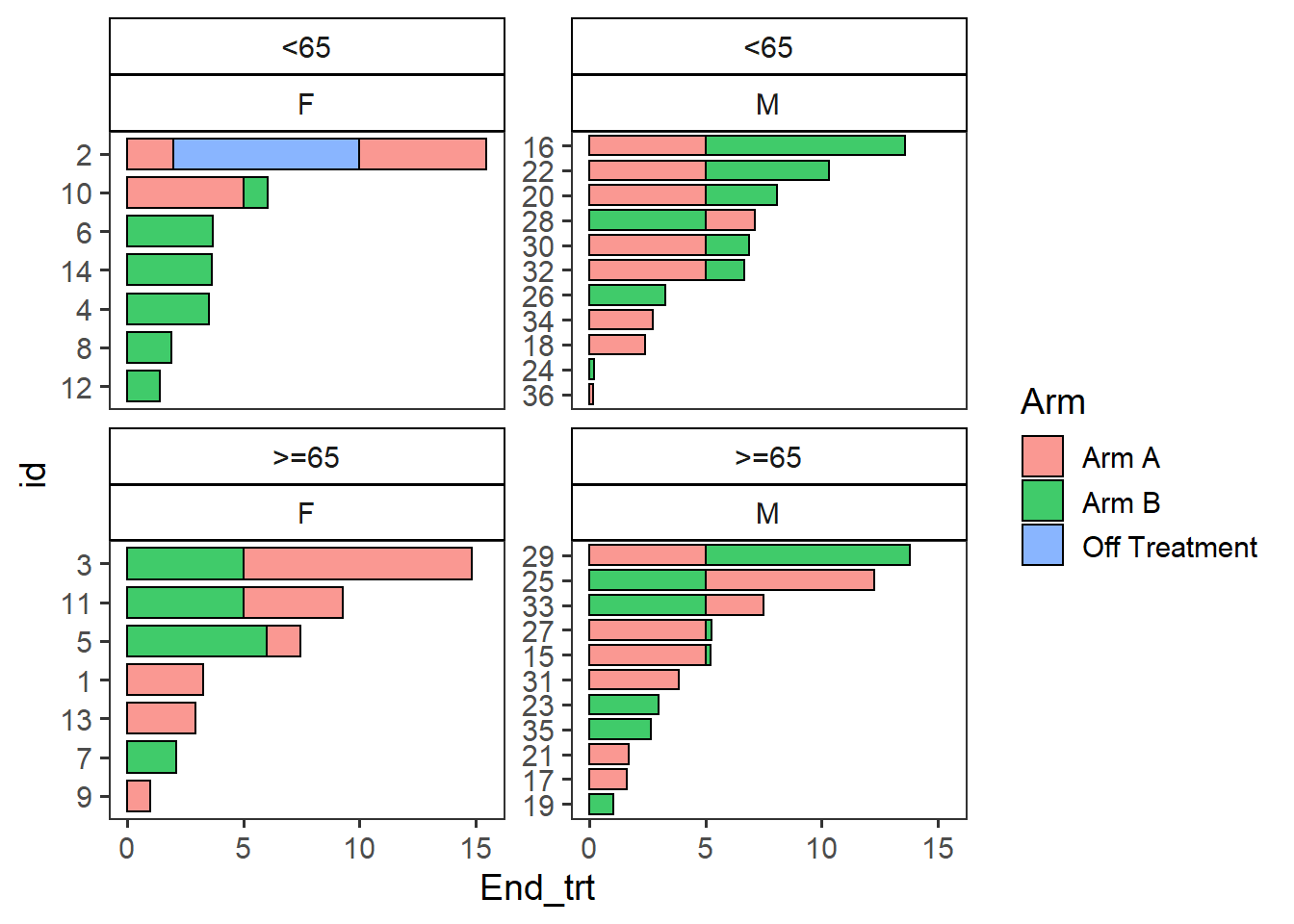• Points are added with the swimmer_points() function
• The required arguments are a dataframe, an id column name, and the column name of the point’s location
• The shape, size, fill, stroke, and transparency can all be mapped to columns
• The argument adj.y can be used to adjust the height position of points withing a bar
• Other aesthetics can be manipulated using geom_point() arguments
AE_plot <- arm_plot + swimmer_points(df_points=
ClinicalTrial.AE,id='id',time='time',name_shape =
'event',size=2.5,fill='white',col='black')
AE_plot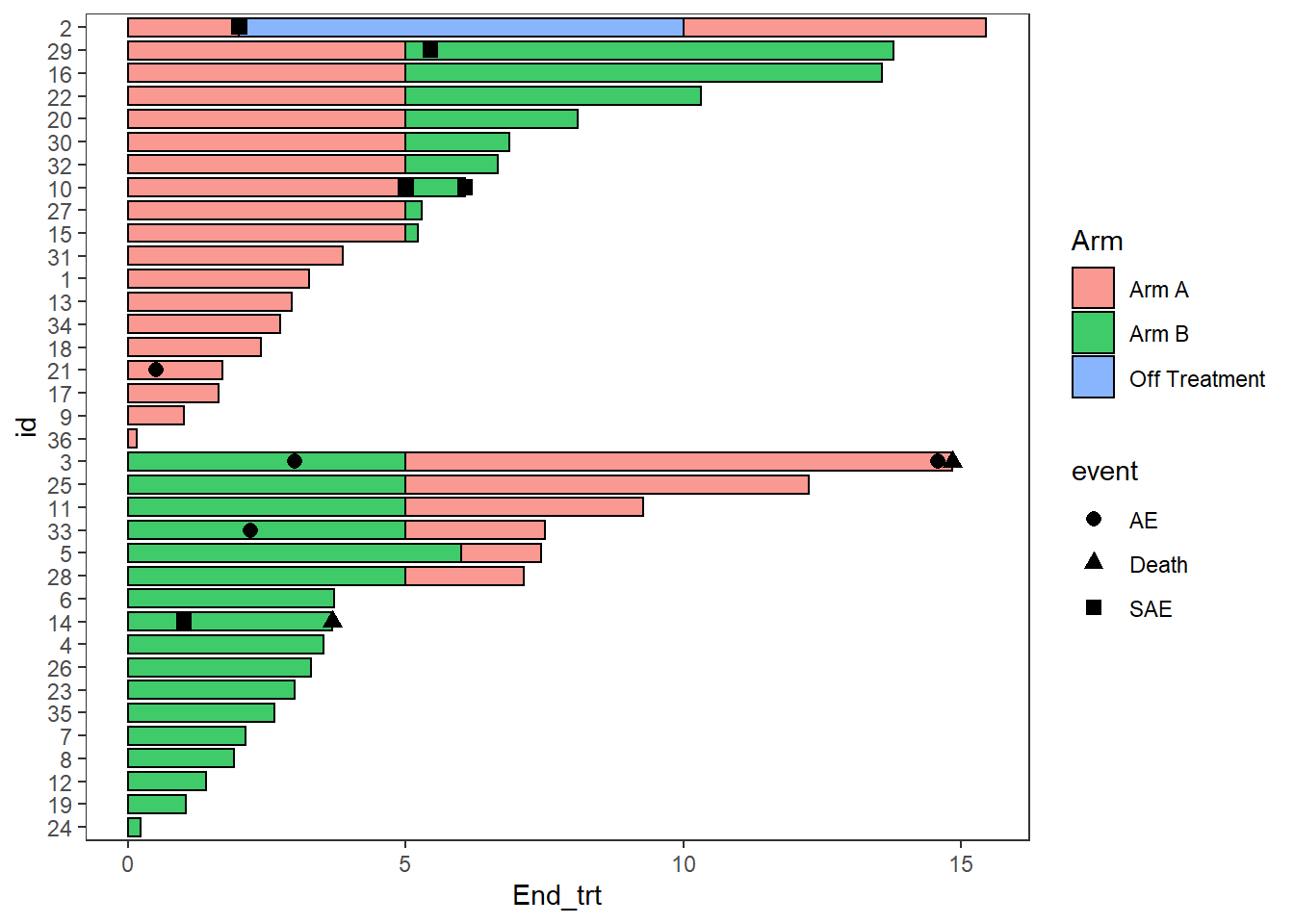Multiple aesthetics can be mapped to different columns

arm_plot + swimmer_points(df_points=
ClinicalTrial.AE,id='id',time='time',name_shape =
'event',size=2.5,fill='white',name_col = 'Related')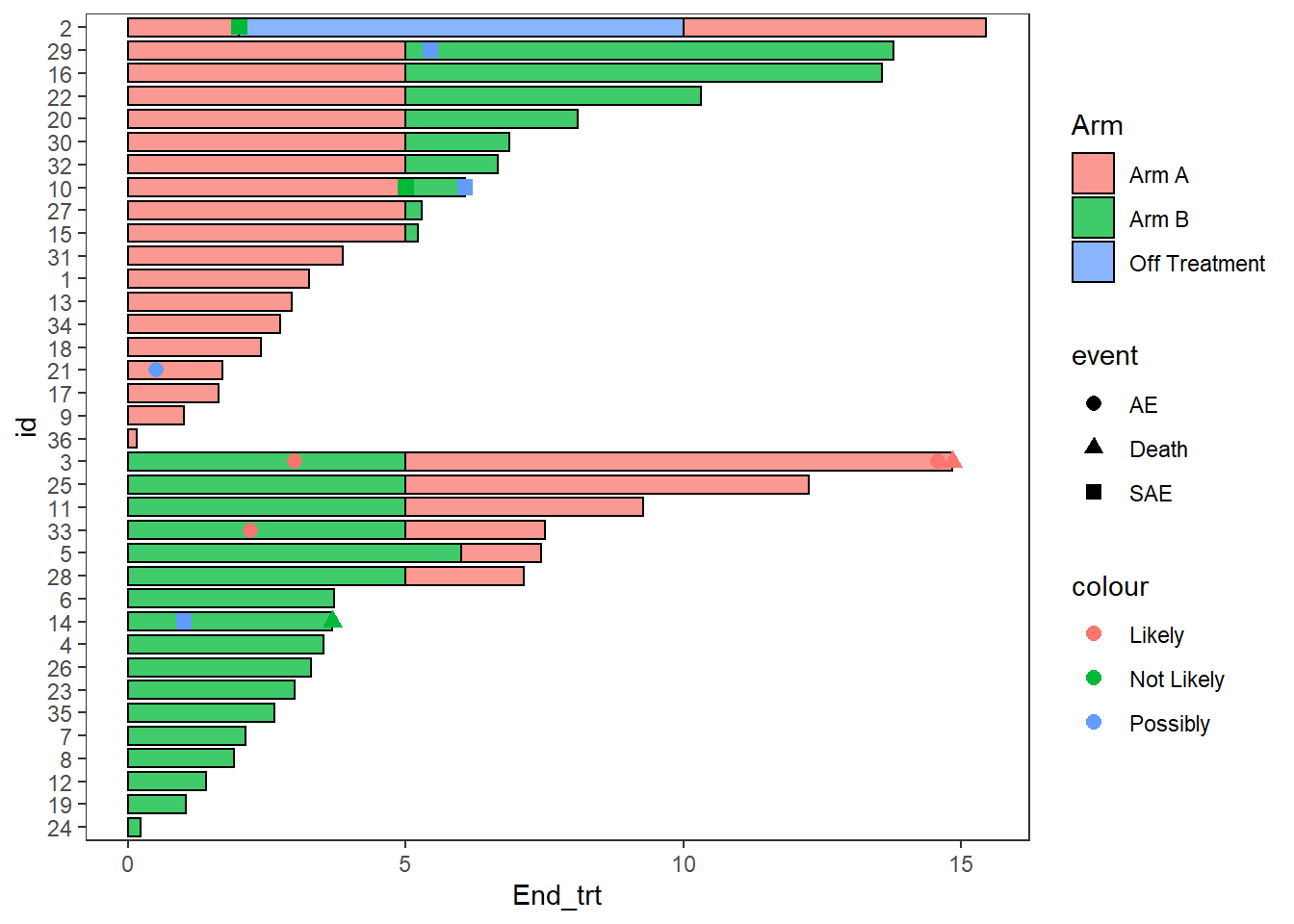• Lines are added with the swimmer_lines() function
• The required arguments are a dataframe, an id column name, and the column names of the line’s start and end locations
• The linetype, colour, size, and transparency can all be mapped to columns
• The argument adj.y can be used to adjust the height position of lines withing a bar
• Other aesthetics can be manipulated using geom_segment() arguments
Response_plot <- arm_plot +
swimmer_lines(df_lines=ClinicalTrial.Response,id='id',start =
'Response_start',end='Response_end',name_col='Response',size=1)

Response_plot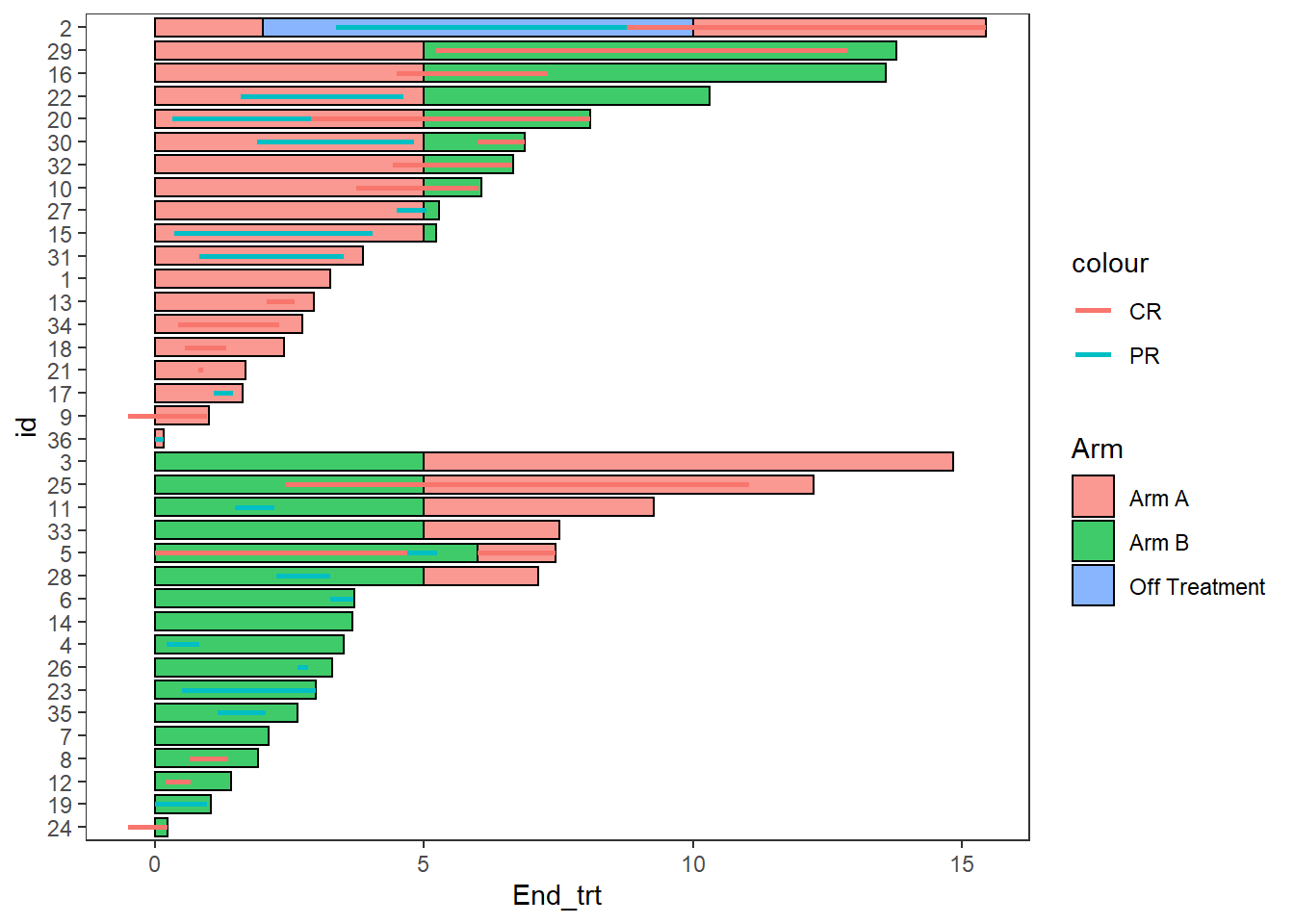• The function swimmer_points_from_lines() adds points to a plot at the start and end of each line
• The required arguments are the same as swimmer_lines()
• An additional argument “cont” can be used to specify lines which do not end
• Other aesthetics can be manipulated using geom_point() arguments
Response_plot_with_points <- Response_plot+
swimmer_points_from_lines(df_lines=ClinicalTrial.Response,id='id',start =
'Response_start',end = 'Response_end', cont =
'Continued_response',name_col='Response',size=2)

Response_plot_with_points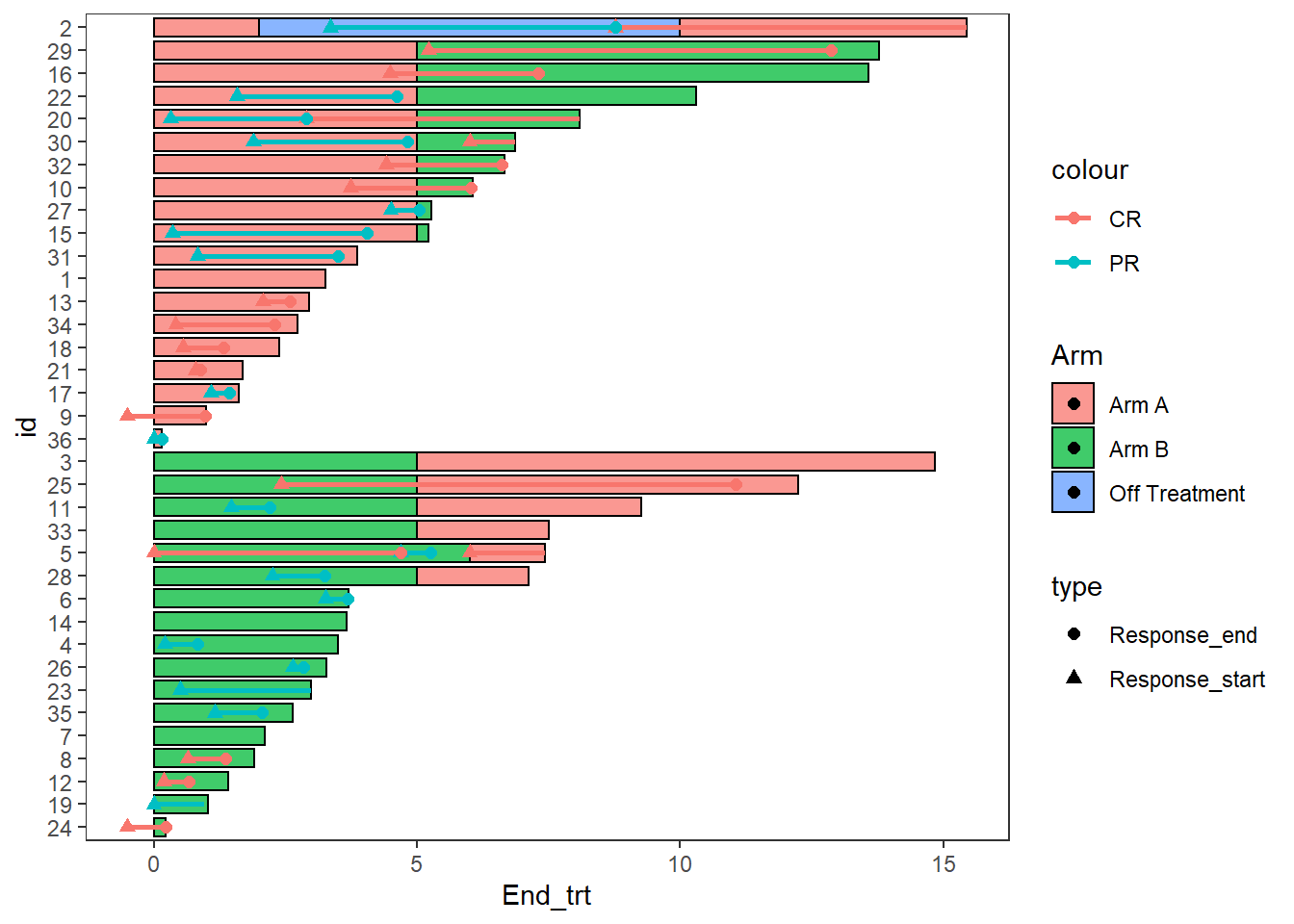• Arrows are added at the end of bars with the swimmer_arrows() function
• The required arguments are a dataframe, an id column name, and the column names of the location the arrows begin
• An additional argument “cont” can be used if only some bars will have an arrow
• The colour can be mapped to a column
• Features of the arrows which can be modified include the size, length and type of arrow
• Other aesthetics can be manipulated using geom_segment() arguments

The example below uses arrows to demonstrate patients remaining on treatment after the end of follow up

AE_plot+
swimmer_arrows(df_arrows=ClinicalTrial.Arm,id='id',arrow_start='End_trt',
cont = 'Continued_treatment',name_col='Arm',type =
"open",cex=1)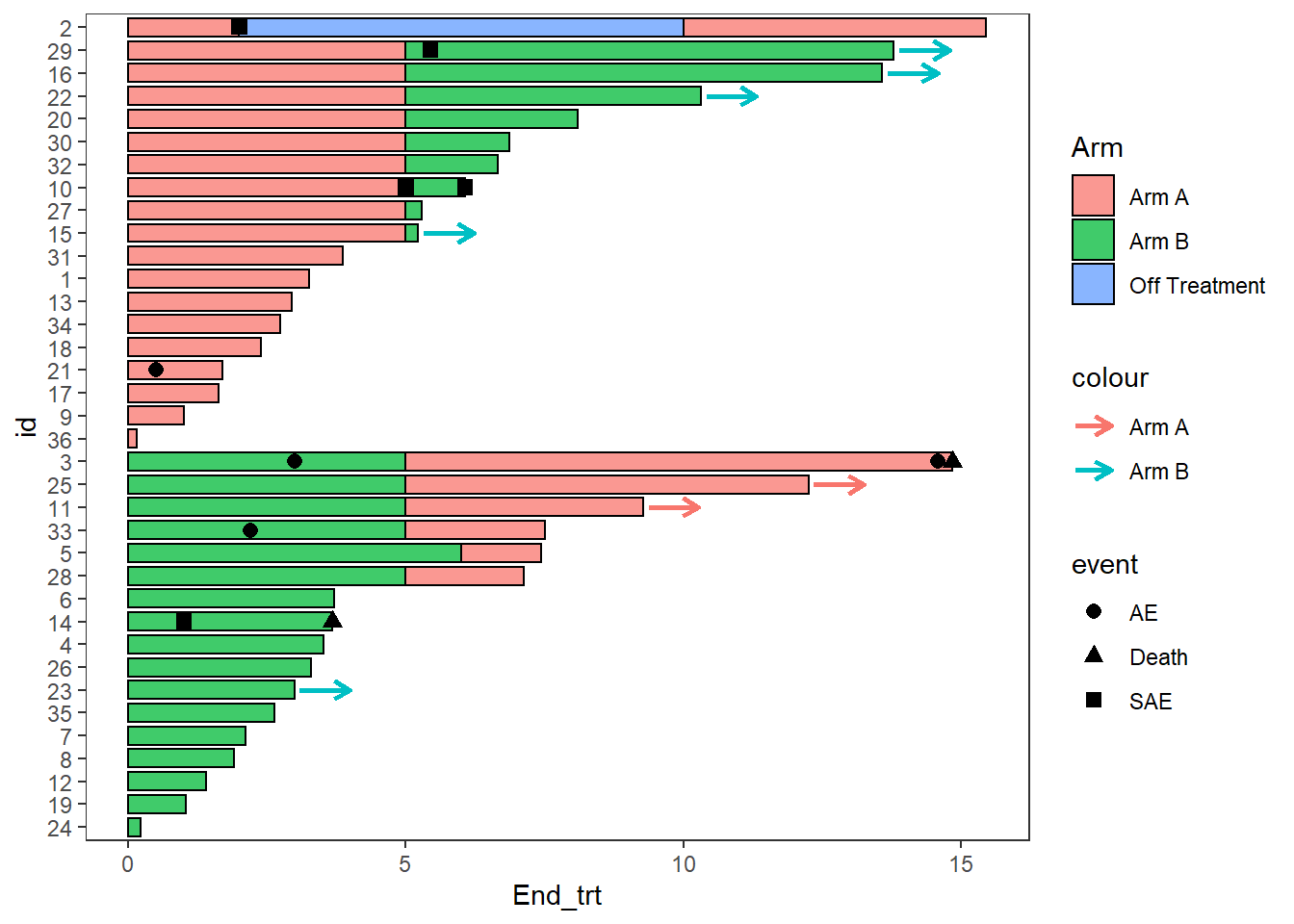Since none of the patients continue on “Off treatment” the arrow colours do not match the bars, this can be fixed by adding the layer scale_color_discrete(drop=FALSE), the option show.legend = FALSE can also be employed as the arrow legend is not necessary

AE_plot <- AE_plot+
swimmer_arrows(df_arrows=ClinicalTrial.Arm,id='id',arrow_start='End_trt',
cont = 'Continued_treatment',name_col='Arm',show.legend = FALSE,type =
"open",cex=1) + scale_color_discrete(drop=FALSE)

AE_plot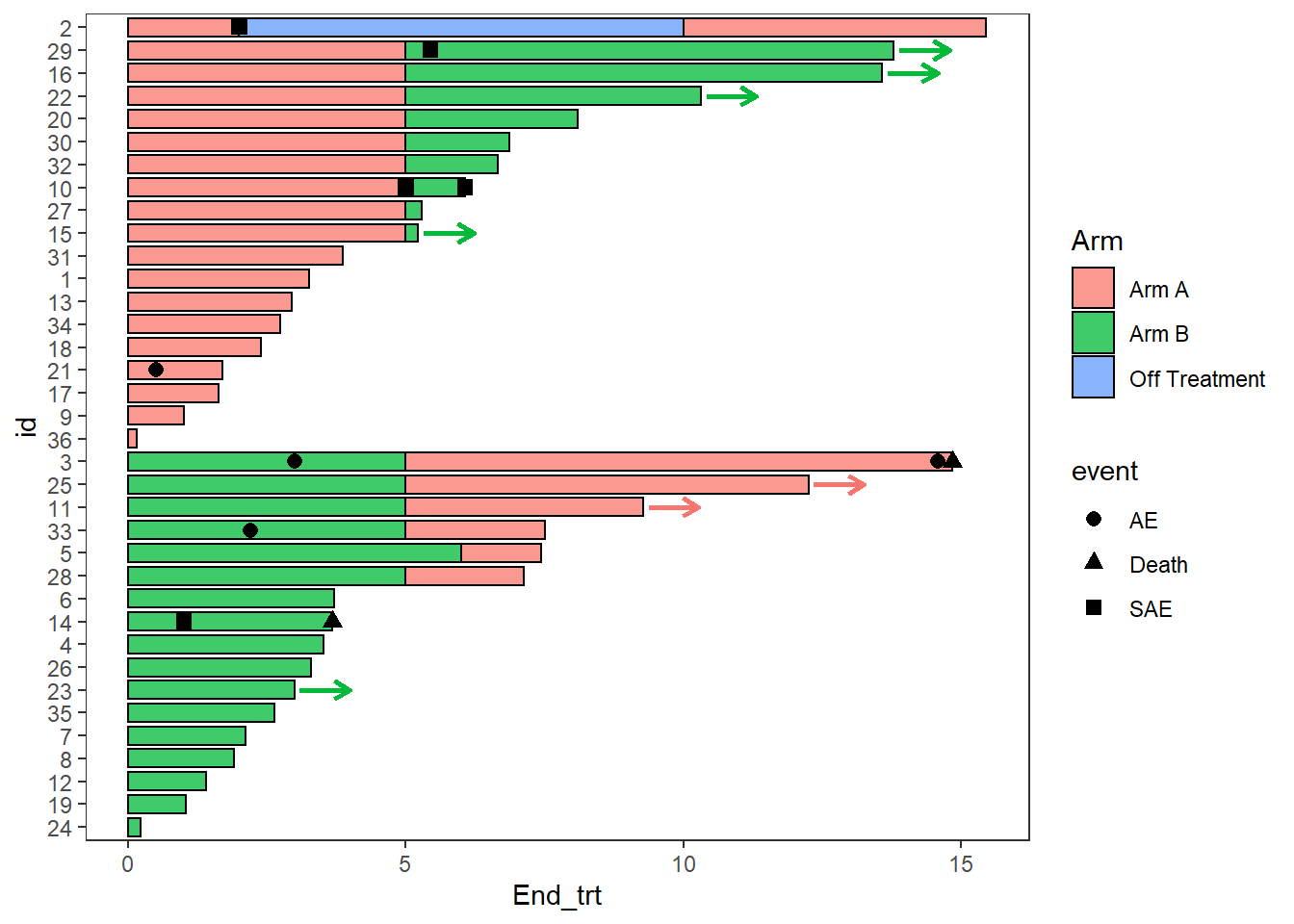Another arrow example, here the arrows are also used to demonstrate a continued treatment

Response_plot_with_points <- Response_plot_with_points+
swimmer_arrows(df_arrows=ClinicalTrial.Response,id='id',arrow_start='Response_end',
cont = 'Continued_response',name_col='Response',show.legend = FALSE,type =
"open",cex=1)

Response_plot_with_points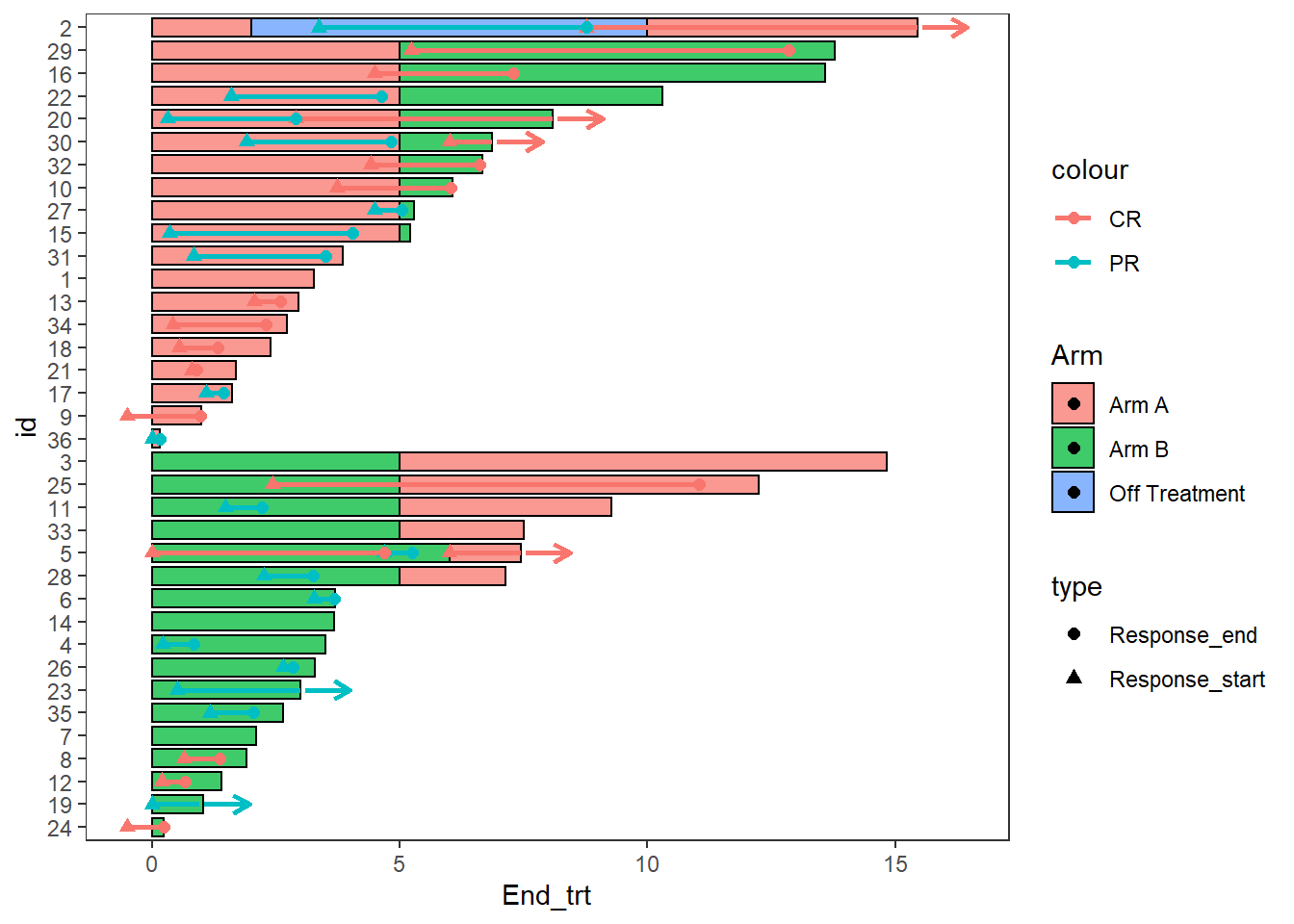Making the plots more aesthetically pleasing with ggplot manipulations

Modifying Colours and shapes

AE_plot <-  AE_plot +
scale_fill_manual(name="Treatment",values=c("Arm A" = "#e41a1c", "Arm B"="#377eb8","Off Treatment"='#4daf4a'))+
scale_color_manual(name="Treatment",values=c("Arm A"="#e41a1c", "Arm B" ="#377eb8","Off Treatment"='#4daf4a')) +

AE_plot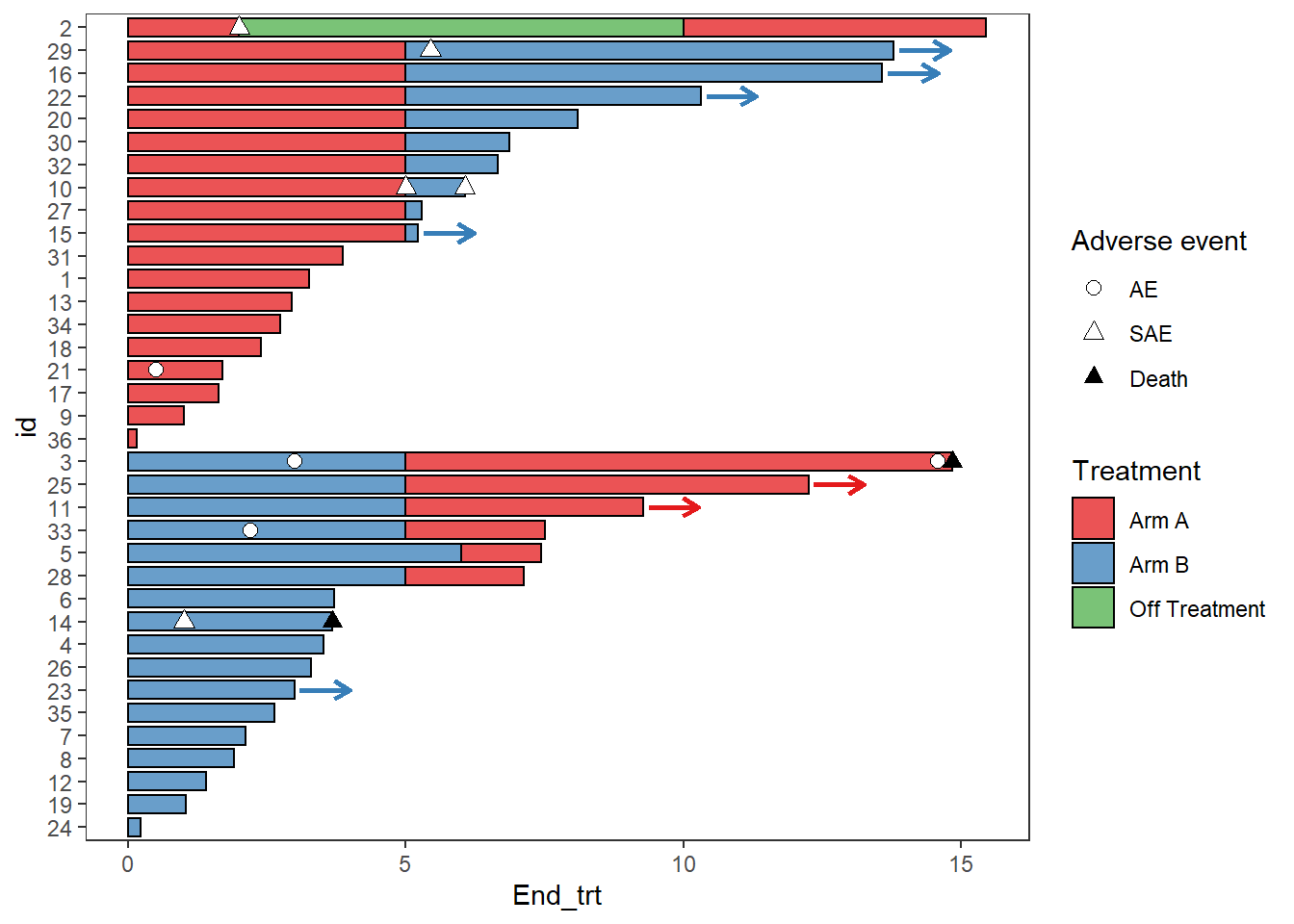Response_plot_with_points <- Response_plot_with_points +
scale_fill_manual(name="Treatment",values=c("Arm A" ="#e41a1c", "Arm B"="#377eb8","Off Treatment"='#4daf4a'))+
scale_color_manual(name="Response",values=c("grey20","grey80"))+
scale_shape_manual(name='',values=c(17,15),breaks=c('Response_start','Response_end'),
labels=c('Response start','Response end'))

Response_plot_with_points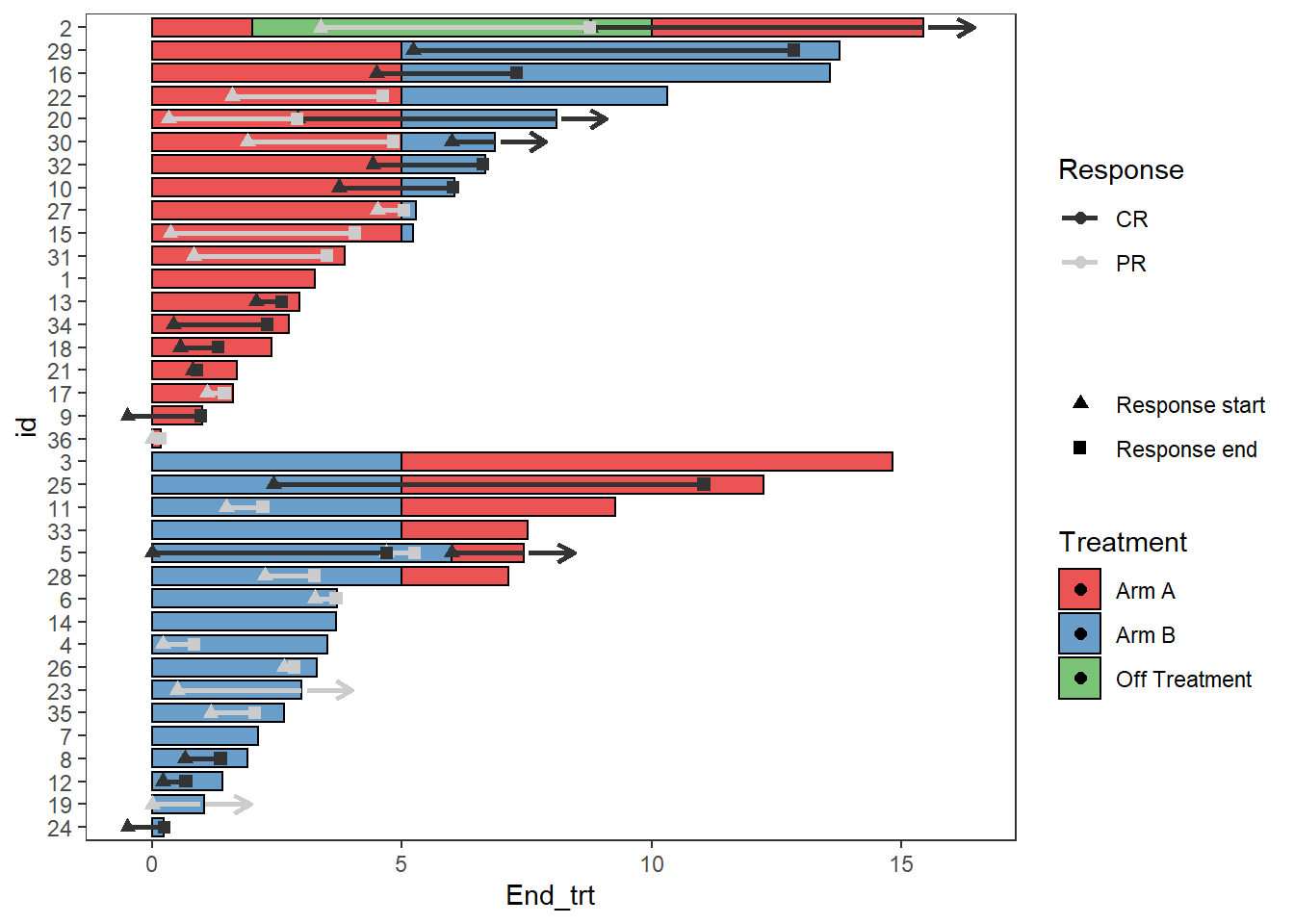Legends

Sometimes there will be points within the fill of the legend, this can be turned off with the layer guides()

Response_plot_with_points <- Response_plot_with_points+guides(fill = guide_legend(override.aes = list(shape = NA)))
Response_plot_with_points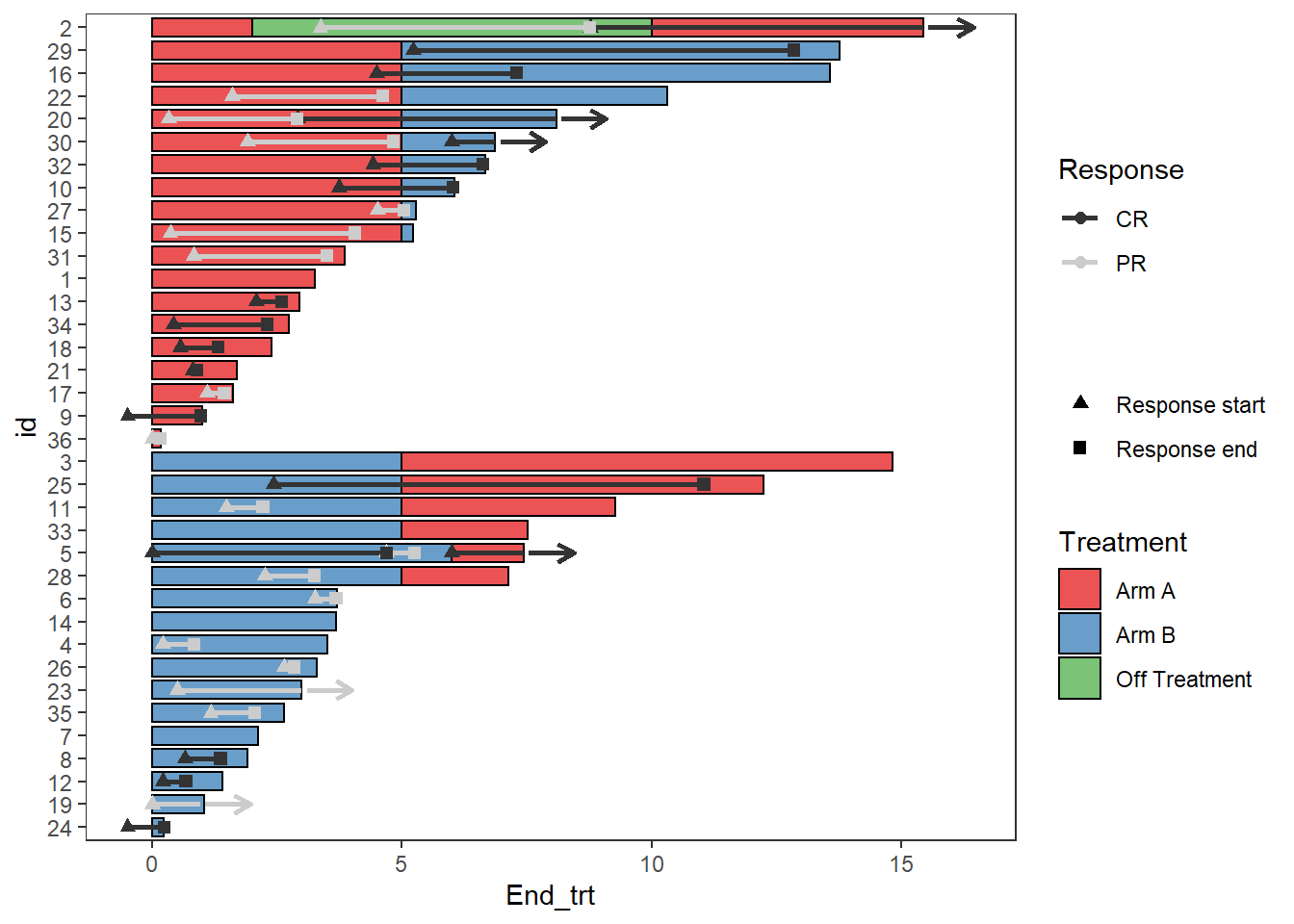A work around to add arrows to the legend is using the symbol for an arrow within annotate()

Response_plot_with_points <- Response_plot_with_points+
annotate("text", x=3.5, y=20.45, label="Continued response",size=3.25)+
annotate("text",x=2.5, y=20.25, label=sprintf('\u2192'),size=8.25)+
coord_flip(clip = 'off', ylim = c(0, 17))
Response_plot_with_points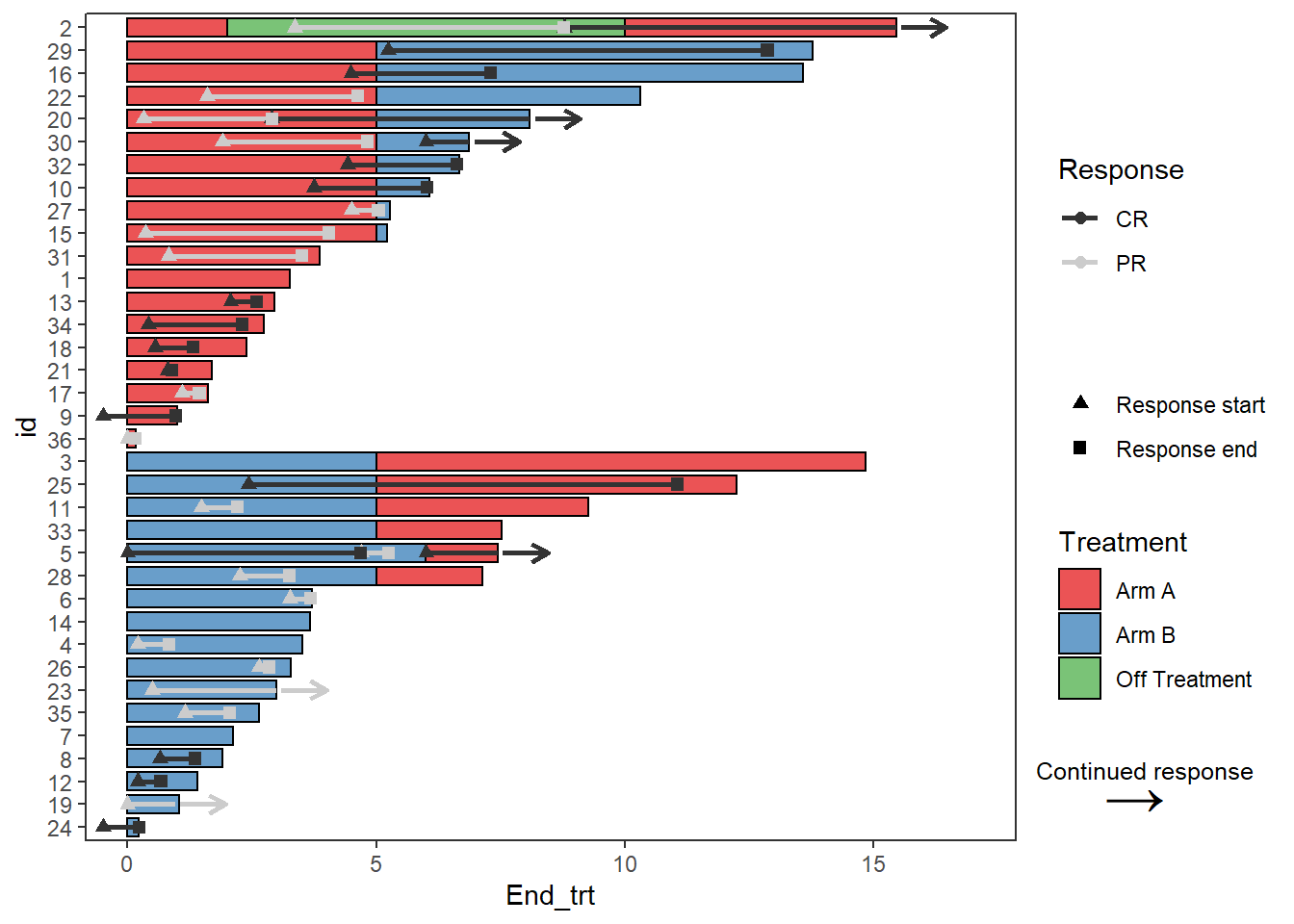axis

The swimmer plot is a bar plot that has been turned on its side, so to modify the x axis it is actually required to change the y axis. This is also the case for adding axis labels

Response_plot_with_points +  scale_y_continuous(name = "Time since enrollment (months)",breaks = seq(0,18,by=3))